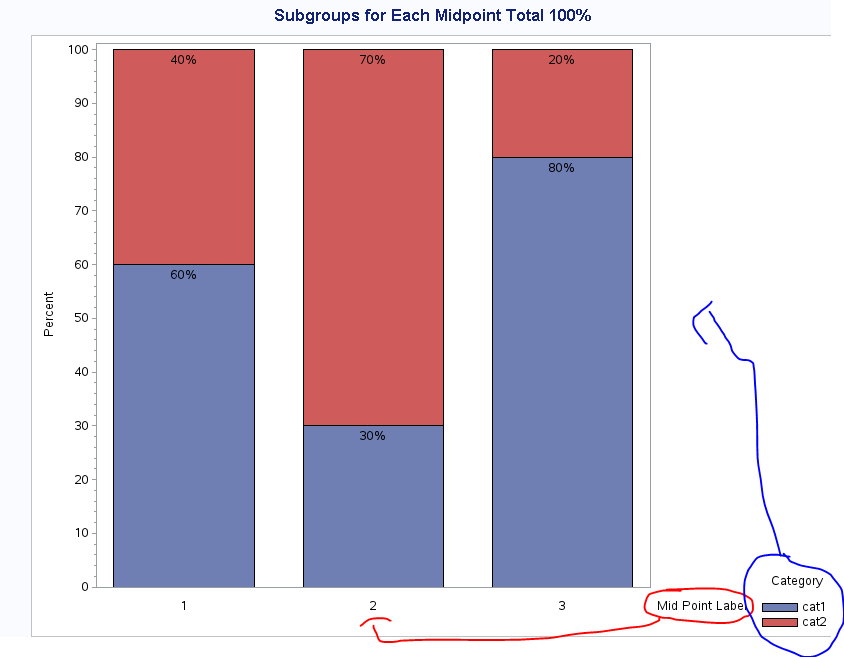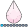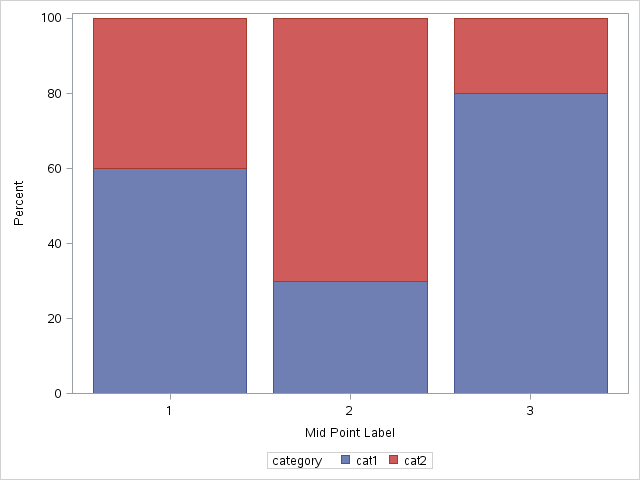## Center axis label along horizontal axis (and legend along vertical axis)

Hi -

I am trying to center the horizontal axis label (along the axis) and center the legend along the vertical axis with proc gchart in this example but with no luck ...

Code modified from here:  http://support.sas.com/kb/41/976.html

``/* Set the graphics environment */ goptions reset=all cback=white border htitle=12pt htext=10pt;  /* Create sample data */ data test;  input midpnt category \$ count;  datalines; 1 cat1 60 1 cat2 40 2 cat1 30 2 cat2 70 3 cat1 80 3 cat2 20 ; run;  /* Define a title for the graph */ title1 'Subgroups for Each Midpoint Total 100%';  /* Suppress the group axis label and values */ axis1 label=none value=none;  /* Angle the label for the response axis */ axis2 label=(angle=90 'Percent'); /* midpoint axis */axis3 label=(position=middle "Mid Point Label");  /* Define legend characteristics */ legend1 label=(position=top justify=center 'Category') position=(center right outside) mode=reserve across=1;  proc gchart data=test;  vbar midpnt / discrete subgroup=category  group=midpnt g100 nozero  freq=count type=percent  inside=percent width=20  gaxis=axis1 raxis=axis2 maxis=axis3  legend=legend1; run; quit;      ``Any help much appreciated!d

4 REPLIES 4djrisks
Barite | Level 11

## Re: Center axis label along horizontal axis (and legend along vertical axis)

Hi,

Do you need to show the percentages in the barchart, and does the legend have to be centered vertically? If not here is a quick solution.

``````proc sgplot data = test;
vbar midpnt / response = count group = category;
xaxis label = "Mid Point Label";
yaxis label = "Percent";
run;``````djrisks
Barite | Level 11

## Re: Center axis label along horizontal axis (and legend along vertical axis)

This code, will also help you to get your percentage labels if you want them. It works in SAS 9.4. There may be an easier way in proc gchart to rearrange the labels and the legend but I am not that familiar with proc gchart,

``````data test2;
set test;
by midpnt category;
retain stacked_result;
if first.midpnt then stacked_result = count;
else stacked_result = stacked_result + count;
length perc \$4;
perc = cats(stacked_result, "%");
stacked_final = stacked_result - (0.05 * stacked_result);
run;

proc sgplot data = test2;
vbarparm category = midpnt response = count / group = category groupdisplay = stacked;
scatter x = midpnt y = stacked_final / markerchar=perc;
xaxis label = "Mid Point Label";
yaxis label = "Percent";
run;``````

## Re: Center axis label along horizontal axis (and legend along vertical axis)

Thanks Djrisks and sorry I wasn't more specific in the original post. I did actually have a solution using sgplot. But I wanted to know how to do it in proc gchart. Trying to compare the two methods. .djrisks
Barite | Level 11

## Re: Center axis label along horizontal axis (and legend along vertical axis)

That's fine @mduarte. I'm sure someone else will help you with Proc gchart then. @Reeza might now.

Thanks.

Discussion stats
• 4 replies
• 1459 views
• 0 likes
• 2 in conversation